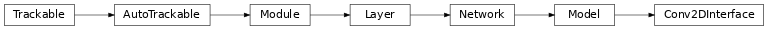# Conv2DInterface¶

Inheritance Diagramclass ashpy.models.convolutional.interfaces.Conv2DInterface[source]

Bases: tensorflow.python.keras.engine.training.Model

Primitive Interface to be used by all ashpy.models.

Methods

 Primitive Interface to be used by all ashpy.models. call(inputs[, training, return_features]) Execute the model on input data.

Attributes

 activity_regularizer Optional regularizer function for the output of this layer. dtype dynamic inbound_nodes Deprecated, do NOT use! Only for compatibility with external Keras. input Retrieves the input tensor(s) of a layer. input_mask Retrieves the input mask tensor(s) of a layer. input_shape Retrieves the input shape(s) of a layer. input_spec Gets the network’s input specs. layers losses Losses which are associated with this Layer. metrics Returns the model’s metrics added using compile, add_metric APIs. metrics_names Returns the model’s display labels for all outputs. name Returns the name of this module as passed or determined in the ctor. name_scope Returns a tf.name_scope instance for this class. non_trainable_variables non_trainable_weights outbound_nodes Deprecated, do NOT use! Only for compatibility with external Keras. output Retrieves the output tensor(s) of a layer. output_mask Retrieves the output mask tensor(s) of a layer. output_shape Retrieves the output shape(s) of a layer. run_eagerly Settable attribute indicating whether the model should run eagerly. sample_weights state_updates Returns the updates from all layers that are stateful. stateful submodules Sequence of all sub-modules. trainable trainable_variables Sequence of variables owned by this module and it’s submodules. trainable_weights updates variables Returns the list of all layer variables/weights. weights Returns the list of all layer variables/weights.
__init__()[source]

Primitive Interface to be used by all ashpy.models.

Declares the self.model_layers list.

static _get_layer_spec(initial_filers, filters_cap, input_res, target_res)[source]

Compose the layer_spec, the building block of a convolutional model.

The layer_spec is an iterator. Every element returned is the number of filters to learn for the current layer. The generated sequence of filters starts from initial_filters and halve/double the number of filters depending on the input_res and target_res. If input_res > target_res the number of filters increases, else it decreases. The progression is always a power of 2.

Parameters
Yields

int – Number of filters to use for the conv layer.

Examples

# Encoder
class T(Conv2DInterface):
pass
spec = T._get_layer_spec(
initial_filers=16,
filters_cap=128,
input_res=(512, 256),
target_res=(32, 16)
)
print([s for s in spec])

spec = T._get_layer_spec(
initial_filers=16,
filters_cap=128,
input_res=(28, 28),
target_res=(7, 7)
)
print([s for s in spec])

# Decoder
spec = T._get_layer_spec(
initial_filers=128,
filters_cap=16,
input_res=(32, 16),
target_res=(512, 256)
)
print([s for s in spec])

spec = T._get_layer_spec(
initial_filers=128,
filters_cap=16,
input_res=(7, 7),
target_res=(28, 28)
)
print([s for s in spec])

[32, 64, 128, 128]
[32, 64]
[64, 32, 16, 16]
[64, 32]


Notes

This is useful since it enables us to dynamically redefine models sharing an underlying architecture but with different resolutions.

call(inputs, training=True, return_features=False)[source]

Execute the model on input data.

Parameters
Returns

tf.Tensor – The model output.